Next: Moments of the Distribution Up: Plasma Fluid Theory Previous: Plasma Fluid Theory

# Introduction

In plasma fluid theory, a plasma is characterized by a few local parameters--such as the particle density, the kinetic temperature, and the flow velocity--the time evolution of which are determined by means of fluid equations. These equations are analogous to, but generally more complicated than, the equations of hydrodynamics.

Plasma physics can be viewed formally as a closure of Maxwell's equations by means of constitutive relations: i.e., expressions for the charge density,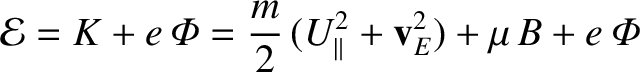, and the current density,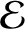, in terms of the electric and magnetic fields,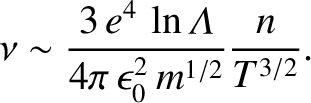and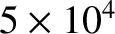. Such relations are easily expressed in terms of the microscopic distribution functions,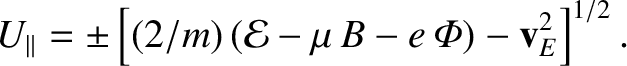, for each plasma species. In fact,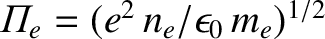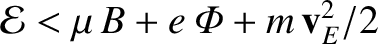(166)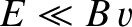(167)

Here,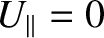is the exact, microscopic'' phase-space density of plasma species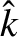(charge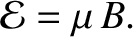, mass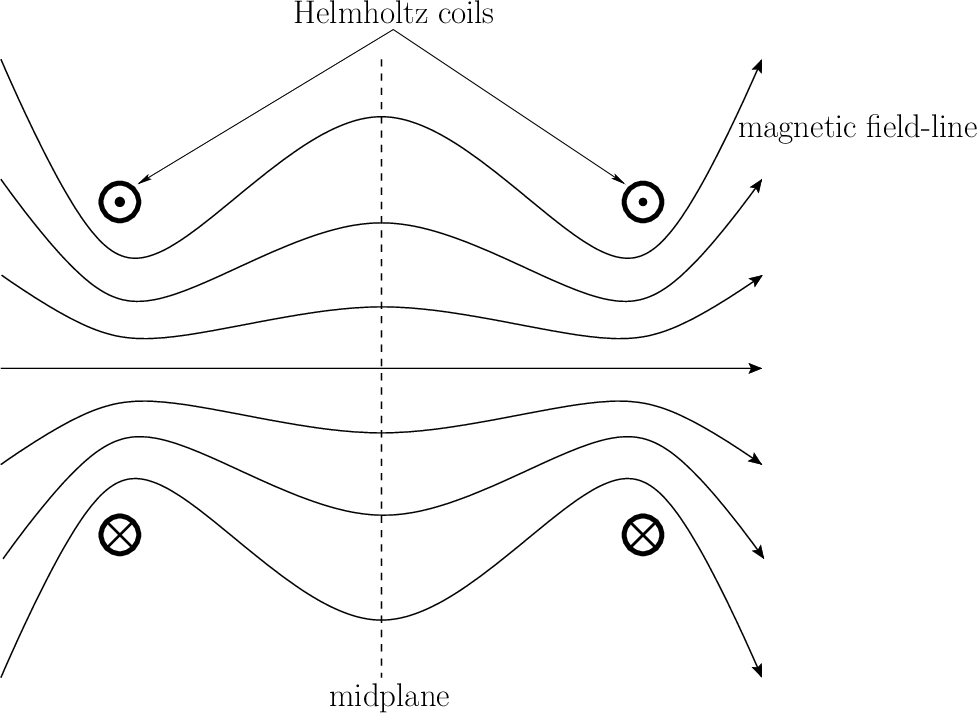) near point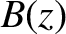at time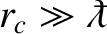. The distribution functionis normalized such that its velocity integral is equal to the particle density in coordinate space. Thus,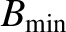(168)

where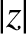is the number (per unit volume) of species-particles near point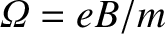at time.

If we could determine eachin terms of the electromagnetic fields, then Eqs. (166)-(167) would immediately give us the desired constitutive relations. Furthermore, it is easy to see, in principle, how each distribution function evolves. Phase-space conservation requires that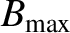(169)

where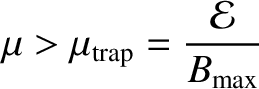is the velocity space grad-operator, and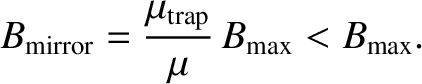(170)

is the species-particle acceleration under the influence of theandfields.

It would appear that the distribution functions for the various plasma species, from which the constitutive relations are trivially obtained, are determined by a set of rather harmless looking first-order partial differential equations. At this stage, we might wonder why, if plasma dynamics is apparently so simple when written in terms of distribution functions, we need a fluid description of plasma dynamics at all. It is not at all obvious that fluid theory represents an advance.

The above argument is misleading for several reasons. However, by far the most serious flaw is the view of Eq. (169) as a tractable equation. Note that this equation is easy to derive, because it is exact, taking into account all scales from the microscopic to the macroscopic. Note, in particular, that there is no statistical averaging involved in Eq. (169). It follows that the microscopic distribution functionis essentially a sum of Dirac delta-functions, each following the detailed trajectory of a single particle. Furthermore, the electromagnetic fields in Eq. (169) are horribly spiky and chaotic on microscopic scales. In other words, solving Eq. (169) amounts to nothing less than solving the classical electromagnetic many-body problem--a completely hopeless task.

A much more useful and tractable equation can be extracted from Eq. (169) by ensemble averaging. The average distribution function,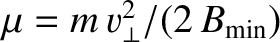(171)

is sensibly smooth, and is closely related to actual experimental measurements. Similarly, the ensemble averaged electromagnetic fields are also smooth. Unfortunately, the extraction of an ensemble averaged equation from Eq. (169) is a mathematically challenging exercise, and always requires severe approximation. The problem is that, since the exact electromagnetic fields depend on particle trajectories,andare not statistically independent of. In other words, the nonlinear acceleration term in Eq. (169),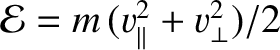(172)

involves correlations which need to be evaluated explicitly. In the following, we introduce the short-hand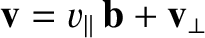(173)

The traditional goal of kinetic theory is to analyze the correlations, using approximations tailored to the parameter regime of interest, and thereby express the average acceleration term in terms of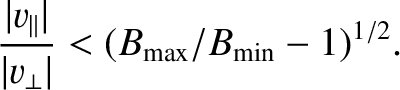and the average electromagnetic fields alone. Let us assume that this ambitious task has already been completed, giving an expression of the form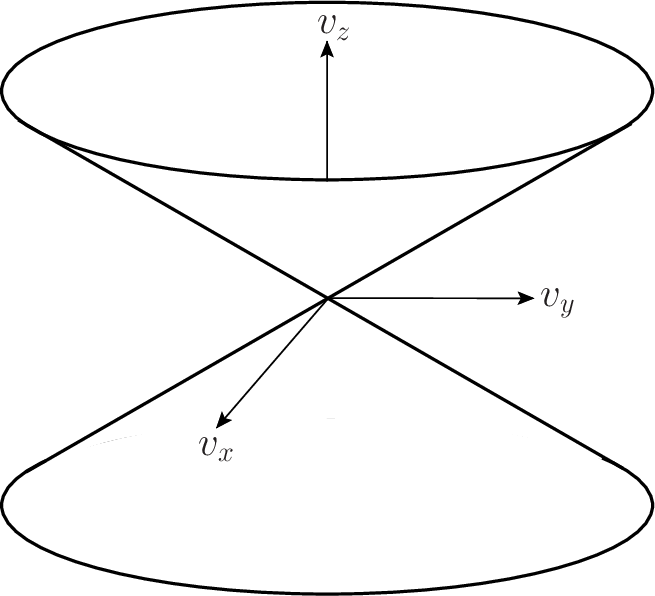(174)

where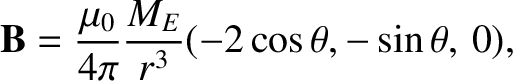is a generally extremely complicated operator which accounts for the correlations. Since the most important correlations result from close encounters between particles,is called the collision operator (for species). It is not necessarily a linear operator, and usually involves the distribution functions of both species (the subscript in the argument ofis omitted for this reason). Hence, the ensemble averaged version of Eq. (169) is written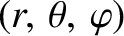(175)

In general, the above equation is very difficult to solve, because of the complexity of the collision operator. However, there are some situations where collisions can be completely neglected. In this case, the apparent simplicity of Eq. (169) is not deceptive. A useful kinetic description is obtained by just ensemble averaging this equation to give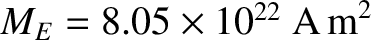(176)

The above equation, which is known as the Vlasov equation, is tractable in sufficiently simple geometry. Nevertheless, the fluid approach has much to offer even in the Vlasov limit: it has intrinsic advantages that weigh decisively in its favour in almost every situation.

Firstly, fluid equations possess the key simplicity of involving fewer dimensions: three spatial dimensions instead of six phase-space dimensions. This advantage is especially important in computer simulations.

Secondly, the fluid description is intuitively appealing. We immediately understand the significance of fluid quantities such as density and temperature, whereas the significance of distribution functions is far less obvious. Moreover, fluid variables are relatively easy to measure in experiments, whereas, in most cases, it is extraordinarily difficult to measure a distribution function accurately. There seems remarkably little point in centering our theoretical description of plasmas on something that we cannot generally measure.

Finally, the kinetic approach to plasma physics is spectacularly inefficient. The species distribution functionsprovide vastly more information than is needed to obtain the constitutive relations. After all, these relations only depend on the two lowest moments of the species distribution functions. Admittedly, fluid theory cannot generally computeandwithout reference to other higher moments of the distribution functions, but it can be regarded as an attempt to impose some efficiency on the task of dynamical closure.Next: Moments of the Distribution Up: Plasma Fluid Theory Previous: Plasma Fluid Theory
Richard Fitzpatrick 2011-03-31